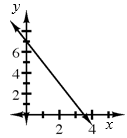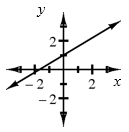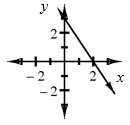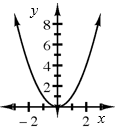### Home > A2C > Chapter 1 > Lesson 1.1.1 > Problem1-7

1-7.

In this course, you will learn shortcuts that allow you to sketch many different types of graphs quickly and accurately. However, when the directions ask you to graph an equation or to draw a graph, this means it is not just a sketch you should do quickly. You need to:

• Use graph paper.

• Label key points.

• Plot points accurately.

On separate sets of axes, graph each of the following equations. If you do not remember any shortcuts for graphing, you can always make an $x → y$ table.

1. $y = −2x + 7$

Graph the y-intercept first. (0, 7)

Notice the NEGATIVE slope.1. $y=\frac{3}{5}x+1$

Remember the slope is RISE OVER RUN.

$\frac{3}{5}$1. $3x+2y=6$

Solve for y before graphing.

$y=-\frac{3}{2}x+3$1. $y=x^2$

Try making a table. Remember to use negative numbers.